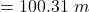Question

A toy radio-controlled car races around a circular track in a time of 2 MINUTES, with a speed of 5.25 m/s. What is the radius of the
circular track? (will give brainliest)

1.The radius will be “100.31 m”. A further solving is given below.

Explanation:

The given values are:

Time,

T = 2 minutes

i.e.,

= 120 seconds

Speed,

V = 5.25 m/s

As we know

⇒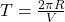then,

⇒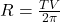On substituting the given values, we get

⇒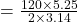⇒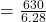⇒